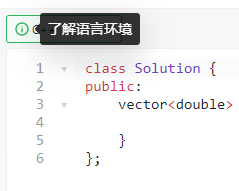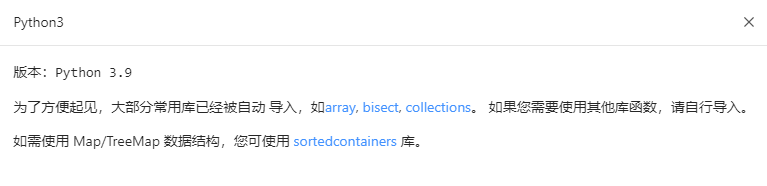## 往期链接

• Week 1 主要内容：数组、二分查找、并查集。

• Week 2 主要内容：数组、字符串、KMP 和字典树。

## 问题汇总

LC1438 可以用 PriorityQueue 吗？不提及是有啥弊端吗？

LC888 sumA - sumB 这个结果万一是奇数是不是答案就不准确了？

LC888 这个直接 sum()int 不会超出范围吗。。

int i = 0; int j = 0; int s = 0;
while(i<A.length||j<B.length){
if(j==B.length||(s<=0&&i!=A.length)){
s+=A[i]; i++; continue;
}
s-=B[j]; j++;
}


LC424 直接返回 right - left 也过了……不知道是哪里会出问题

LC480 Python 暴力解法居然不超时？永远的神

Python 不公平的点在于, 虽然它语言解释运行很慢, 但一些底层库 ( list 等) 却基本都是用 CC++ 实现的, 所以快一些(相对于不用库,直接类c数组操作而言),但是 Python 时限又高(8-10s), 所以总体来说相当于用 C++ 写了个暴力又放宽了时间限制,成就了暴力的奇迹。

P.S. [x:x] = [v] 确实要比 insert 要快，因为 [x:x] = [v] 的底层实现调用 memmove 库函数来搬运插入之后的元素，而 insert 采用 for 循环搬运元素，参考 list 源码

LC480 有点不理解，如果优先队列中有需要延迟删除的数，那么此时堆顶中的数还是中位数吗？为啥多出了数之后中间还是中位数啊？

1. 堆顶元素必定有效
2. 两个堆的元素数目不包含延迟删除的元素

LC480 JavaPriorityQueue 不是自带 remove(Object o) 删除任意元素的方法吗？为什么还要写延迟删除呢？

JavaPriorityQueueremove(Object o) 方法的时间复杂度是 $O(n)$ （虽然删除的复杂度是 $O(logn)$ , 但在二叉堆中搜索指定元素的复杂度是线性的）而不是 $O(logn)$, 在数据范围较大的情况下很可能无法通过。（为什么？link

LC1208 这里为什么要求连续？

LC1423 为什么我用递归/记忆化搜索/动态规划做超时了？

## 学习建议

### 双指针

• ITMO Academy: pilot course - Two Pointers Method CF EDU

Codeforces 平台的教学课程最新推出的一个章节，虽然定位是竞赛课程，但是对新手友好。同时也附有从易到难的练习题目供巩固，且因为 Judge 有语言时间补正，因此对于 Python 选手相对友好。

### 关于 LC480 滑动窗口中位数 的一点延伸和个人总结

• 每滑动一次均排序计算中位数： 滑动次数为 $n-k$, 每次排序时间复杂度为 $O(k\log k)$, 因而总时间复杂度为 $O((n - k)klog k) = O(nk\log k)$.

• 每滑动一次均线性选择计算中位数：滑动次数为 $n-k$, 每次线性选择（类似快排，忘记了？看这里）时间复杂度为 $O(k)$, 因而总时间复杂度为 $O((n - k)k) = O(nk)$.

• 用单数组来维护有序结构，每次二分插入与删除：滑动次数为 $n-k$, 每次二分查找时间复杂度为 $O(\log k)$, 数组插入删除任意元素时间复杂度为 $O(k)$, 因而总时间复杂度为 $O((n - k)k) = O(nk)$.

• *转化为静态区间第 k 小问题，离散化后用线段树/可持久化线段树（主席树）求解，时间复杂度为 $O((n - k)\log n) = O(n\log n)$. link1 link2

• 带有「删除指定元素」的双堆：滑动次数为 $n-k$, 堆的大小为 $O(k)$, 每次插入元素复杂度为 $O(\log k)$, 每次保持平衡操作（弹出堆顶插入另一堆）复杂度为 $O(\log k)$, 每次删除指定元素复杂度为 $O(k)$ （想想为什么？），因而总时间复杂度为 $O((n - k)k) = O(nk)$.

• 事实上，通过一些额外的操作，可以降低「删除指定元素」操作的时间复杂度，使其不再成为瓶颈，进而使得总时间复杂度为 $O(n\log k)$. 读者可以思考一下如何实现。
• 带有「延迟/惰性删除」的双堆：滑动次数为 $n-k$, 由于「延迟/惰性删除」，堆的大小最大可能为为 $O(n)$, 每次插入元素复杂度为 $O(\log n)$, 每次保持平衡操作（弹出堆顶插入另一堆 + 若堆顶需要删除则删除）复杂度为 $O(\log n)$, 因而总时间复杂度为 $O((n - k)\log n) = O(n\log n)$.

• 用双平衡树代替双堆：滑动次数为 $n-k$, 对于平衡树，由于不存在「延迟/惰性删除」造成的额外大小增加，因此平衡树的大小为 $O(k)$, 平衡树增删操作复杂度均为 $O(\log k)$, 因而总时间复杂度为 $O((n - k)\log k) = O(n\log k)$.

PS: 对数时间删除指定元素的二叉堆是可以做到的，仅需要手动建立并维护元素和堆中索引的双向映射，便可以使单次「找到指定元素」的复杂度降低至 $O(1)$, 进而使得「删除指定元素」操作的时间复杂度为 $O(k)$, 与平衡树一致。但是一般编程语言中的优先队列/二叉堆均不支持此操作，因此需要手动实现二叉堆。同时，建立维护索引的操作虽然代码量较少，但是会增加一定的调试难度，一般情况还是建议使用平衡树代替。（感谢 @OTTFF 指出）

• (C++) 单 multiset , 每次ss.begin() 更新 （包括但不限于 std::advance() ）中位数迭代器（此处时间复杂度为 $O(k)$ ），总时间复杂度为 $O(nk)$.

• (C++) 单 multiset , 手动维护中间位置的迭代器，每次至多移动一步（此处诸多细节从略），总时间复杂度为 $O(n\log k)$, 与最优解一致。

• (C++) 使用 pb_ds 库的 tree 数据结构（红黑树实现）, 使用 find_by_order() 方法维护中位数，总时间复杂度为 $O(n\log k)$, 与最优解一致。 link.

• (Java) 双 PriorityQueue , 使用 remove(Object o) 方法（此处时间复杂度见前文）而非延迟删除，总时间复杂度为 $O(nk)$.

• (Python) 单 SortedList, 采用下标访问中位数，总时间复杂度可以认为 $O(n\log k)$, 与最优解一致。

PS1: 很多人使用了 Pythonbisect.insort() 或者 [x:x] = [v], 此处操作复杂度依旧应当视为线性，但由于硬件和软件的因素（参见前文），其实际运行时间可能并不逊于 SortedList，因而在 LeetCode 的评测系统下有能力作为一种合理的过题方案。但是，在可能出现的复杂度分析场合（尤其是面试），依旧要注意。

PS2: 使用有序数据结构（可以对数时间进行某些操作）时，需要注意某些看似简化思考和代码量的操作可能会不必要地增加时间复杂度，从而降低对应数据结构选取的意义。

## 关于语言环境### 简单介绍 Python 的 sortedcontainers 库

• SortedList link

• SortedSet link

• SortedDict link

• __init__(iterable = None, key = None) 初始化，支持自定义初始迭代器和比较器。时间复杂度 $O(n\log n)$.

• __contains__(value) ss.__contains__(value) == value in ss 判断是否存在。时间复杂度 $O(1)$.

• __len__() ss.__len()__ == len(ss) 返回长度。

• add(value) 加入元素。时间复杂度大致 $O(\log n)$.

• remove(value) / discard(value) 删除单个值对应的元素。注意 remove 不存在的元素会报错。时间复杂度大致 $O(\log n)$.

• __getitem__(index) ss.__getitem__(index) == ss[index] 按下标访问，支持切片操作。时间复杂度大致 $O(\log n)$.

• __delitem__(index) ss.__delitem__(index) == del ss[index] 删除下标对应的元素，支持切片操作，下标不存在会报错。时间复杂度大致 $O(\log n)$.

• pop(index = -1) 删除下标对应的元素，下标不存在会报错。时间复杂度大致 $O(\log n)$.

• bisect_left(value) / bisect_right(value) 二分查找下标，与 bisect 库对应的方法基本一致。时间复杂度大致 $O(\log n)$.

31

2

2

1

1

1